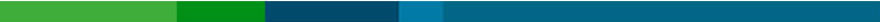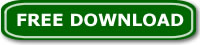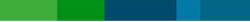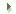|   Home    |  Download    |  Buy Now    |  Support    | |  Contact Us    |# Filters

 Notch Notch Filter Band Pass Band Pass Filter High Pass High Pass Filter Low Pass Low Pass Filter High Shelf High Shelf Filter Low Shelf Low Shelf Filter FFT FFT Filter Peak EQ Peak EQ Filter

About the audio filters (this section is for the advanced users):

All filter transfer functions were derived from analog prototypes (that
are shown below for each filter type) and had been digitized using the
Bilinear Transform (Waveview). BLT frequency warping has been taken into account
for both significant frequency relocation and for bandwidth readjustment.

First, given a biquad transfer function defined as:

b0 + b1*z^-1 + b2*z^-2
H(z) =
a0 + a1*z^-1 + a2*z^-2

This shows 6 coefficients instead of 5 so, depending on your architecture,
you will likely normalize a0 to be 1 and perhaps also b0 to 1 (and collect
that into an overall gain coefficient). Then your transfer function would
look like:

(b0/a0) + (b1/a0)*z^-1 + (b2/a0)*z^-2
H(z) =
1 + (a1/a0)*z^-1 + (a2/a0)*z^-2

or

1 + (b1/b0)*z^-1 + (b2/b0)*z^-2
H(z) = (b0/a0) *
1 + (a1/a0)*z^-1 + (a2/a0)*z^-2

The most straight forward implementation would be the Direct I form (second equation):

y[n] = (b0/a0)*x[n] + (b1/a0)*x[n-1] + (b2/a0)*x[n-2] - (a1/a0)*y[n-1] - (a2/a0)*y[n-2]

This is probably both the best and the easiest method to implement in the 56K.

Now, given:

sampleRate (the sampling frequency)

frequency ("wherever it's happenin', man." "center" frequency
or "corner" (-3 dB) frequency, or shelf midpoint frequency,
depending on which filter type)

dBgain (used only for peaking and shelving filters)

bandwidth in octaves (between -3 dB frequencies for BPF and notch
or between midpoint (dBgain/2) gain frequencies for peaking EQ)

_or_ Q (the Electrical Engineering kind of definition)

_or_ S, a "shelf slope" parameter (for shelving EQ only). when S = 1,
the shelf slope is as steep as it can be and remain monotonically
increasing or decreasing gain with frequency.

First compute a few intermediate variables:

A = sqrt[ 10^(dBgain/20) ]
= 10^(dBgain/40) (for peaking and shelving EQ filters only)

omega = 2*PI*frequency/sampleRate

sin = sin(omega)
cos = cos(omega)

alpha = sin/(2*Q) (if Q is specified)
= sin*sinh[ ln(2)/2 * bandwidth * omega/sin ] (if bandwidth is specified)

beta = sqrt[ (A^2 + 1)/S - (A-1)^2 ] (for shelving EQ filters only)

Then compute the coefs for whichever filter type you want:

The analog prototypes are shown for normalized frequency.
The bilinear transform substitutes:

1 1 - z^-1
s <- *
tan(omega/2) 1 + z^-1

LPF: H(s) = 1 / (s2 + s/Q + 1)

b0 = (1 - cos)/2
b1 = 1 - cos
b2 = (1 - cos)/2
a0 = 1 + alpha
a1 = -2*cos
a2 = 1 - alpha

HPF: H(s) = s2 / (s2 + s/Q + 1)

b0 = (1 + cos)/2
b1 = -(1 + cos)
b2 = (1 + cos)/2
a0 = 1 + alpha
a1 = -2*cos
a2 = 1 - alpha

BPF (constant skirt gain): H(s) = s / (s2 + s/Q + 1)

b0 = Q*alpha
b1 = 0
b2 = -Q*alpha
a0 = 1 + alpha
a1 = -2*cos
a2 = 1 - alpha

BPF (constant peak gain): H(s) = (s/Q) / (s2 + s/Q + 1)

b0 = alpha
b1 = 0
b2 = -alpha
a0 = 1 + alpha
a1 = -2*cos
a2 = 1 - alpha

Notch: H(s) = (s2 + 1) / (s2 + s/Q + 1)

b0 = 1
b1 = -2*cos
b2 = 1
a0 = 1 + alpha
a1 = -2*cos
a2 = 1 - alpha

PeakingEQ: H(s) = (s2 + s*(A/Q) + 1) / (s2 + s/(A*Q) + 1)

b0 = 1 + alpha*A
b1 = -2*cos
b2 = 1 - alpha*A
a0 = 1 + alpha/A
a1 = -2*cos
a2 = 1 - alpha/A

LowShelf: H(s) = A * (s2 + beta*s + A) / (A*s2 + beta*s + 1)

b0 = A*[ (A+1) - (A-1)*cos + beta*sin ]
b1 = 2*A*[ (A-1) - (A+1)*cos ]
b2 = A*[ (A+1) - (A-1)*cos - beta*sin ]
a0 = (A+1) + (A-1)*cos + beta*sin
a1 = -2*[ (A-1) + (A+1)*cos ]
a2 = (A+1) + (A-1)*cos - beta*sin

HighShelf: H(s) = A * (A*s2 + beta*s + 1) / (s2 + beta*s + A)

b0 = A*[ (A+1) + (A-1)*cos + beta*sin ]
b1 = -2*A*[ (A-1) + (A+1)*cos ]
b2 = A*[ (A+1) + (A-1)*cos - beta*sin ]
a0 = (A+1) - (A-1)*cos + beta*sin
a1 = 2*[ (A-1) - (A+1)*cos ]
a2 = (A+1) - (A-1)*cos - beta*sin

## Music Editing MasterMusic Editing MasterAudio Amplifier ProEasy Audio EditorAudio Silence Trimmer ProMP3 File HiderEasy Video Logo RemoverEasy Blurry Video ClearerFull Video Audio MixerEasy Video ReverserEasy Video Sync FixerVideoDetach ProEasy Shaky Video FixerEasy Video Fisheye FixerMusic Editing Master Help
 How to Start Wave View Top Tool Bar Bottom Tool Panel Left Tool Panel Marker Panel File Folder Panel Edit Audio File Trim or Join Audio Files Handle Audio Channels Rip Audio from Audio CD Extract Audio from Video File Convert Text to Speech Merge Audio with Video File Record Sound Edit Audio Tag Batch Convert Audio Formats Burn Audio CD Burn Data DVD or CD Function List (Menu Items) File Edit Operations Filters View Tools Options Audio Editing GlossaryCopyright © 2002-2019 DanDans Digital Media Audio Editing